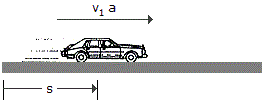# Engineering Mechanics - Kinematics of Particle (KOP) - Discussion

### Discussion :: Kinematics of Particle (KOP) - General Questions (Q.No.10)

10.A car, initially at rest, moves along a straight road with constant acceleration such that it attains a velocity of 60 ft/s when s = 150 ft. Then after being subjected to another constant acceleration, it attains a final velocity of 100 ft/s when s = 325 ft. Determine the average velocity and average acceleration of the car for the entire 325-ft displacement.

 [A]. vavg = 80.0 ft/s, aavg = 15.15 ft/s2 [B]. vavg = 45.2 ft/s, aavg = 13.91 ft/s2 [C]. vavg = 80.0 ft/s, aavg = 12.57 ft/s2 [D]. vavg = 55.0 ft/s, aavg = 15.15 ft/s2

Explanation:

No answer description available for this question.

 Yemane said: (Apr 30, 2016) How to find the acceleration?

 Kumara Swamy said: (Jun 1, 2016) Average acceleration= (vf - vi)/total time. Total time = 5 + 2.1875 = 7.1875. Vf = 100, vi = 0. Acceleration = 100/7.1875 = 13.913.

 Shubham. said: (Jul 3, 2020) How to find the time? Please explain.